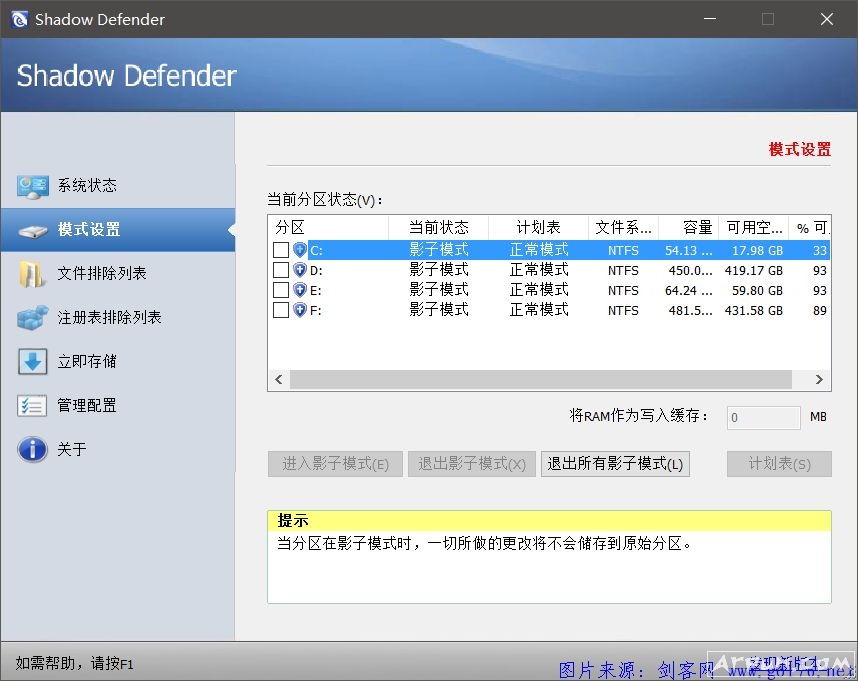# WIN10中文版影子系统 shadow defender 2018出品

• 运行环境：PC-windows操作系统
• 软件语言：简体中文|授权方式：
• 软件类型：国产软件 - 系统工具 - 系统增强
• 软件大小：3.31 MB
• 下载星级：
• 软件官网：暂无
• 更新时间：2018/4/5 11:33:03

【软件截图】【基本介绍】

WIN10还没有兼容的影子系统system。 我们国家内那款WIN10影子系统完全不兼容， 一用就是蓝屏相信都知道那款软件， 所以还是用这款国外的中文版shadow defender完美兼容WIN10， 完全稳定stable使用！

U3XT4-K82R3-KKGYB-8K1NN-CDQI4

PTAHE-MMKNT-QPKTR-ZZ14K-14063

SYYM5-CG87V-FB8JM-8MCEC-M68UN

[translate]
;authorinfo=Simplified Chinese Translation 简体翻译 by: Clarence

[common]

10=系统状态
11=模式设置
12=文件排除列表
13=立即存储
14=管理配置
15=关于
16=注册表排除列表

20=注册(&R)
21=如需帮助，请按F1
22=发现新版本.

31=显示桌面提示(&S)
32=隐藏托盘图标(&I)
33=帮助(&H)
34=退出所有影子模式(&E)

100=影子模式
101=正常模式

200=提示

1000=你没有足够的权限。
1001=操作被终止。
1002=操作已完成。
1003=密码无效。
1004=密码错误。请输入您的密码并重试。
1005=请稍候……
1006=正在储存 %s
1007=储存文件“%s”失败 (%s)。
1008=储存文件“%s”失败，错误代码 (%d)。
1009=正在储存 %s ...，已完成 %d。
1011=所有文件|*.*||
1012=您必须选择至少一个分区。
1013=进入影子模式前，请先储存您未保存的文件并关闭所有其它应用程序。\n\n继续请点击“是”。\n\n注意：影子模式中，在排除列表里的文件和文件夹一切所做的更改将直接储存到原始分区并将不给予任何警告或通知。
1014=由 Shadow Defender 储存
1015=如果分区在影子模式中，储存选定文件和文件夹到原始分区。
1016=%s 空间不足，请立即退出影子模式。
1017=%s 处于影子模式。
1018=%s 处于影子模式。
1019=%s 已存在。\n您确定要替换？
1021=无法打开文件 (%s)。
1023=储存完毕。

10000=系统状态
10001=处于影子模式中的分区：
10002=排除列表(&X)：
10003=当前状态：
10004=容量：
10005=已用空间：
10006=可用空间：
10007=实际可用空间：
10008=SD 所使用空间：
10009=路径
10010=缓存使用情况：
10011=容量：
10012=已用空间：

20000=模式设置
20001=当前分区状态(&V)：
20002=分区
20003=当前状态
20004=文件系统
20005=容量
20006=可用空间
20007=% 可用
20008=计划表

20009=进入影子模式(&E)
20010=退出影子模式(&X)
20011=退出所有影子模式(&L)
20012=当分区在影子模式时，一切所做的更改将不会储存到原始分区。
20013=将所选分区置于影子模式。
20014=将所选分区退出影子模式。
20015=放弃在影子模式下一切的所做的更改并将所有分区退出影子模式。
20016=分区中某些文件还在使用中，建议先关闭使用中的文件以达到最好保护。\n\n欲继续请点击“确定”。
20017=计划表(&S)
20018=下次重启时是否将分区添加到影子模式。
20019=将RAM作为写入缓存：
20020=当写入缓存被启用，所有的更改将会写入内存。\n警告：如果内存较小，不要启用该选项。

30000=文件排除列表
30001=您想要从影子保护分区中排除的文件和文件夹(&X)：
30002=路径
30003=添加文件(&F)
30004=添加文件夹(&L)
30005=删除(&D)
30006=应用(&P)
30007=把您想从影子模式里排除的文件或文件夹添加到以上列表，然后点击“应用”按钮。当使用这些文件或文件夹，所有的更改将被保存到原始分区。
30008=请选择一个您要添加到排除列表的文件夹：
30009=储存排除列表为文件
30010=从文件加载排除列表
30012=您选择的文件至少有一个处于影子模式。请在将该分区置于影子模式之前添加文件\n操作被取消。
30013=您选择的文件夹已经处于影子模式。\n操作被取消。

40000=立即储存
40001=您想要储存到原始分区的文件和文件夹(&S)：
40002=路径
40003=添加文件(&F)
40004=添加文件夹(&L)
40005=删除(&D)
40006=应用(&P)
40007=添加文件和文件夹到上述列表中，然后点击“应用”按钮，一切对这些文件和文件夹的所做的更改将储存到原始分区中。 一旦文件和文件夹再次被改变，你需要重新存储它们。
40008=请选择一个您想要储存的文件夹：
40009=储存文件列表为文件
40010=从文件加载文件列表

50000=管理配置
50001=启用托盘图标(&I)
50002=启用桌面提示(&T)
50003=启用右键菜单(&S)
50004=启用密码控制(&W)
50005=设置新密码(&N)
50006=您的密码为空，请设置一个新密码。
50007=新密码设置成功。
50008=通过右键菜单储存文件时需要输入密码。(&C)
50009=保护分区空间不足时提示通知
50010=更多详情(&D)
50011=警告：当保护分区空间不足时，可能导致SD不能正常操作。
50012=随系统启动(&S)
50013=加密写入缓存区(&E)
50014=自动检查更新
50015=影子模式里启用休眠

60000=注册表排除列表
60001=您想要排除的注册表项目(&X)：
60002=项目
60003=添加(&A)
60004=删除(&D)
60005=应用(&P)
60006=添加您想要排除的注册表项目到上面的列表里，然后点击‘应用’按钮。当这些注册表项目被使用时，所做的更改将自动储存到原始分区。
60007=请选择一个您想要添加到排除列表里的注册表项目：
60008=储存排除列表到文件
60009=从文件导入排除列表

70001=请输入密码(&P)：
70002=确定
70003=取消

80000=设置密码
80001=密码(&P)：
80002=确认(&C)：
80003=确定
80004=取消

90000=您的 Shadow Defender 试用版已到期，欲继续使用请先注册。
90001=您的 Shadow Defender 将在 %d 天后到期，请注册。
90002=购买(&B)
90003=稍后(&L)
90004=注册(&R)

100001=请输入产品注册码(&R)：
100002=获取注册码
100003=确定
100004=取消

110001=版权终归 (C) 2007-2012，shadowdefender.com 所有。
110003=版本
110004=用户
110005=组织
110006=序列号
110007=在线查看更多关于 Shadow Defender 的信息
110008=警告：本计算机程序受著作权法和国际公约的保护，未经授权擅自复制或散布本程序的部分或全部内容，将承受严厉的民事和刑事处罚，对已知的违反者将给予法律范围内的全面制裁。
110009=确定

120000=进入影子模式
120001=进入影子模式前，请先储存您的文件。然后点击“确定”。\n\n注意：影子模式中，在排除列表里的文件和文件夹一切所做的更改将直接储存到原始分区并将不给予任何警告或通知。
120002=关机后退出影子模式(&X)
120003=重启后继续影子模式(&E)
120004=
120005=确定
120006=取消

130000=退出影子模式
130001=如果您所选择的分区正在被其他应用程序使用，为使更改生效需重启或关闭计算机。建议退出影子模式之前关闭所有其它应用程序及储存您的文件。继续请点击“确定”。
130002=放弃除排除列表之外的所有更改(&D)
130003=(推荐)
130004=储存所有更改(&C)
130005=
130006=确定
130007=取消
130008=强制卸载分区(&F)
130009=强制卸载分区将可能导致正在使用该分区的应用程序发生不可预料的错误。\n\n您想继续吗？

140001=您所选择的分区无法退出影子模式 (可能某些应用程序正在调用其中文件)，为使更改生效需重启或关闭计算机。\n\n您要重启或关闭计算机吗？(重启或关机后，系统会自动退出影子模式)
140002=为使更改生效需重启或关闭计算机。\n重启或关机后，系统会自动退出影子模式。\n\n您要现在重启或关闭计算机吗？
140003=退出影子模式并重启(&R)
140004=退出影子模式并关机(&S)
140005=稍后重启或关机(&L)
140006=（关机时系统将自动退出影子模式）
140007=确定
140008=取消

150001=警告：此操作将无法撤消。\n您确定要把数据储存到原始分区吗？
150002=储存已删除的文件(&D)
150003=不包括子目录(&R)
150004=确定
150005=取消
150006=只限已删除文件(&O)
150007=暂停
150008=继续

160000=预留空间设置
160001=当保护分区的可用空间少于列表里的预留值，将显示托盘气球信息提示。
160002=点击项目

160003=分区
160004=预留空间 (MB)
160005=确定
160006=取消
160007=其他

170001=继续操作需要验证码，请输入验证码：
170002=提示：
170003=验证码(&V)：
170004=确定
170005=取消
180000=选择一个注册表项目
180001=请选择您要排除的注册表项目：
180002=确定
180003=取消

* 为了达到最快的下载速度，推荐使用迅雷下载
* 请一定升级到最新版WinRAR解压缩软件才能正常解压本站提供的软件!
* 站内提供的所有软件由网上搜集，若侵犯了你的版权利益，敬请来信通知我们!